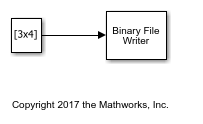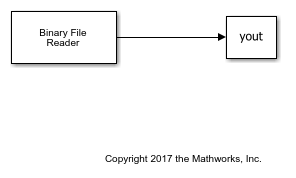Use a Binary File Reader block to read real and complex matrix data from a binary file in a row-major format.

### Write the Data

Write the matrix A = [1 2 3 8; 4 5 6 10; 7 8 9 11] to a binary file, `Matdata.bin` using the Binary File Writer block. The block writes the specified header, `struct('A',[1 2],'B','x7')` followed by the data.

Open the model.

```open_system('writeMatrixData') ```Run the model to write the data to `Matdata.bin`.

```sim('writeMatrixData') ```

The `Binary File Reader` block reads the data in binary file `Matdata.bin` into 4 channels, with each channel containing 5 samples. The File header parameter of the reader specifies the header of the data. If the exact header is not known, you must at least specify the prototype of the header, that is, its size and data type.

Open the model.

```open_system('readMatrixData') ```Run the model to read the data. Display the output data variable, `yout`.

```sim('readMatrixData') display(yout) ```
```yout = 1 2 3 8 4 5 6 10 7 8 9 11 0 0 0 0 0 0 0 0 ```

Each frame of `yout` contains frames of the matrix A, followed by zeros to complete the frame. The original matrix A contains 4 channels with 3 samples in each channel. The reader is specified to read data into 4 channels, with each channel containing 5 samples. Because there are not enough samples to complete the frame, the reader appends zeros at the end of each frame.

If you select the Data is complex parameter, the reader reads the data as an M-by- N matrix of complex values, where M and N are specified by the Samples per frame and Number of channels parameters, respectively. Select the Data is complex parameter and run the model.

```set_param('readMatrixData/Binary File Reader','IsDataComplex','on') sim('readMatrixData') display(yout) ```
```yout = 1.0000 + 2.0000i 3.0000 + 8.0000i 4.0000 + 5.0000i 6.0000 +10.0000i 7.0000 + 8.0000i 9.0000 +11.0000i 0.0000 + 0.0000i 0.0000 + 0.0000i 0.0000 + 0.0000i 0.0000 + 0.0000i 0.0000 + 0.0000i 0.0000 + 0.0000i 0.0000 + 0.0000i 0.0000 + 0.0000i 0.0000 + 0.0000i 0.0000 + 0.0000i 0.0000 + 0.0000i 0.0000 + 0.0000i 0.0000 + 0.0000i 0.0000 + 0.0000i ```

The block reads the data as interleaved real and imaginary components. If there are not enough samples in the binary file to complete the matrix, the reader fills those samples with zeros.

If you make any changes to the model, save the model before closing.

```save_system('readMatrixData') close_system('readMatrixData') close_system('writeMatrixData') ```